# Effectiveness to calculate the outlet temperatures of a heat exchanger

PCal
Homework Statement:
The data below relates to a specific heat exchanger. A reliable colleague has looked up an effectiveness chart and says that the effectiveness in the given operating conditions is 0.82.
For hot fluid: mass flowrate qm= 0.7 kg s -1, specific heat capacity cp=1.8 kJ kg-1 K-1, inlet temp= 140°C . For cold fluid: mass flowrate qm= 0.6 kg s -1, specific heat capacity cp=4.2 kJ kg-1 K-1, inlet temp= 15°C . Area of heat transfer surface=14m^2, Overall heat transfer coefficient 360 W m-2 K-1.
Determine:
i. the two outlet temperatures.
ii. the heat transfer rate.
I've spent ages on this but can't seem to find an equation to find both outlet temperatures! Any help would be greatly appreciated.
Relevant Equations:
ε=(T_HI-T_H2)/(T_HI-T_C1 )
Because the specific heat capacity is lower for the hot fluid:
ε=(T_hi-T_h2)/(T_hi-T_C1 )

0.82=(140-T_h2)/(140-15)

140-T_h2= 102.5

T_h2= 140-102.5=37.5°

I'm not sure if I'm actually on the right track here, if I am this is as far as I've gotten. I can't seem to then find an equation to calculate T_c2 other than the equation which my lessons tell me is only used when the specific heat capacity is lower for the cold fluid.

#### Attachments

•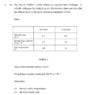Question.jpg
36 KB · Views: 579

PCal
Hi,

I wonder where you got your relevant equation. I am used to a different definition of ##\varepsilon##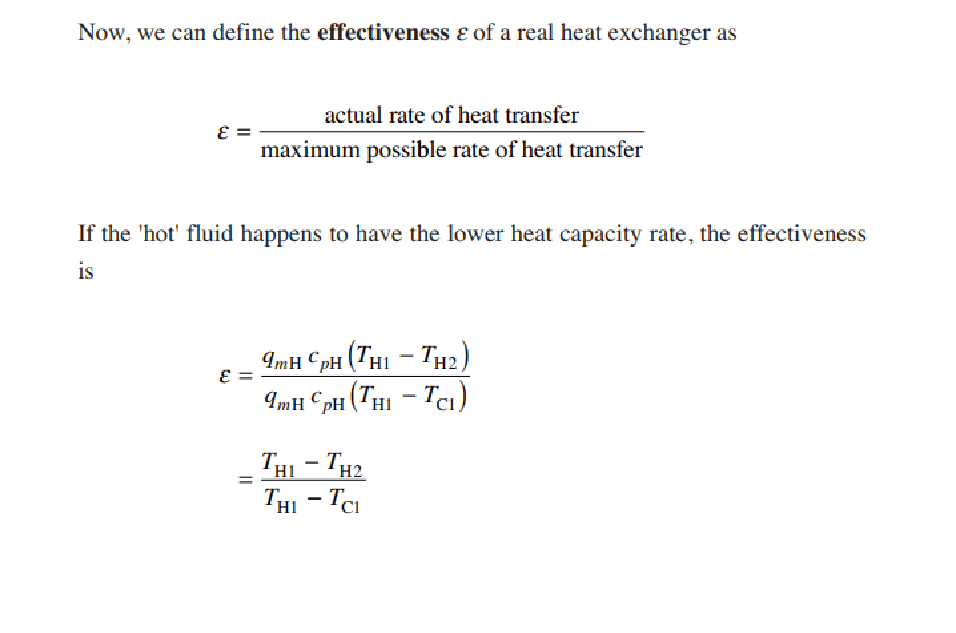In my lessons ε has been used to define effectiveness

PCal
This is where I'm currently at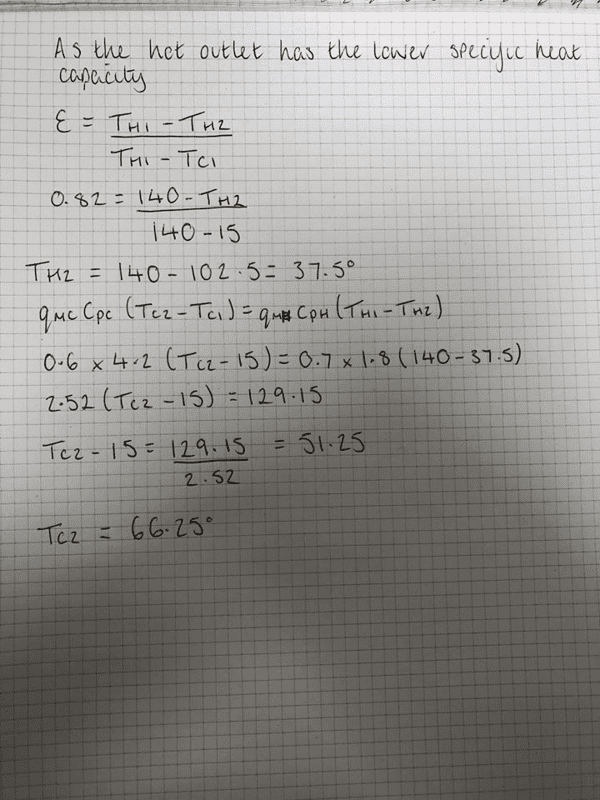Homework Helper
In my lessons ε has been used to define effectiveness
My bad -- long time agoTo make up: Got the same result as you did.

•PCal
PCal
My bad -- long time agoTo make up: Got the same result as you did.
fab thank you!

PCal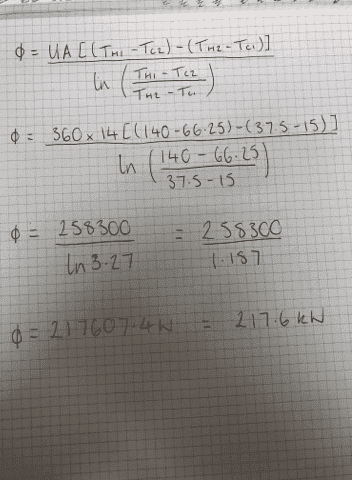one more thing, how's this for the heat transfer rate please?

Mentor
Is this a countercurrent heat exchanger or a co-current heat exchanger? I assume that it is countercurrent, correct?

PCal
Is this a countercurrent heat exchanger or a co-current heat exchanger? I assume that it is countercurrent, correct?
The question doesn't actually specify, I've assumed it's countercurrent/ cross flow

PCal
Is this a countercurrent heat exchanger or a co-current heat exchanger? I assume that it is countercurrent, correct?
Part C of the question also refers to the correction factor which leads me to think it's cross flow

Homework Helper
one more thing, how's this for the heat transfer rate please?
In #1 you calculated 129 kW
##Q_{\rm max} ## = 157.2, so I find 218 kW hard to believe ...

The 218 comes from UA*LMTD which applies to a countercurrent heat exchanger. Here you have a less effective heat transfer configuration, so a higher LMTD and your expression isn't valid.
Must say the exercise composer doesn't make it easy for you. Good you did the UA*LMTD check -- I did it too and had to look more than twiceIs this a countercurrent heat exchanger or a co-current heat exchanger? I assume that it is countercurrent, correct?
Hi Chet! I was kind of struggling with that too. With ##\ C_{\rm min} =1.26,\ ## and ##\ UA =5.04##, NTU = 4. C_r=0.5 so that ##\ \varepsilon## for co-current would be 0.66 and counter 0.93 with the 0.82 in between.

PCal
In #1 you calculated 129 kW
##Q_{\rm max} ## = 157.2, so I find 218 kW hard to believe ...

The 218 comes from UA*LMTD which applies to a countercurrent heat exchanger. Here you have a less effective heat transfer configuration, so a higher LMTD and your expression isn't valid.
Must say the exercise composer doesn't make it easy for you. Good you did the UA*LMTD check -- I did it too and had to look more than twiceHi Chet! I was kind of struggling with that too. With ##\ C_{\rm min} =1.26,\ ## and ##\ UA =5.04##, NTU = 4. C_r=0.5 so that ##\ \varepsilon## for co-current would be 0.66 and counter 0.93 with the 0.82 in between.

Of course, I didn't click onto that! Does 129.5kW sound like a more believable heat transfer rate please? Apologies this modules taking a lot to get my head around!

Mentor
Yes. If I assume counterflow, I get outlet temperatures of 73 for the cold stream and 24.1 for the hot stream.

Homework Helper
Of course, I didn't click onto that! Does 129.5kW sound like a more believable heat transfer rate please? Apologies this modules taking a lot to get my head around!
The 129.15 follows from the given information, yes.

PCal
Yes. If I assume counterflow, I get outlet temperatures of 73 for the cold stream and 24.1 for the hot stream.
Sorry I'm so confused, I got 66.25 for the cold stream outlet and 37.5 for the hot, this seems way off the mark!

Mentor
Sorry I'm so confused, I got 66.25 for the cold stream outlet and 37.5 for the hot, this seems way off the mark!
My calculation was for countercurrent flow. If you check your numbers, the heat transfer rate based on the log mean temperature difference does not match the heat transfer rate based on the flows and heat capacities (if the flow were assume to be countercurrent).

PCal
Ohh that makes sense thank you, I'll look back over my lesson and calculations.

PCal
My calculation was for countercurrent flow. If you check your numbers, the heat transfer rate based on the log mean temperature difference does not match the heat transfer rate based on the flows and heat capacities (if the flow were assume to be countercurrent).
Still completely baffled I'm afraid, it seems you've used 1-e^-ntu(1-c)/1-Ce^-ntu(1-c) to get effectiveness= 0.92 and used that in your equations. Going through the same equation I got the same answer, however my question states that the effectiveness is 0.82. Given that, my outputs of cold= 66.25 and hot=37.5 would be correct wouldn't they?

Mentor
Still completely baffled I'm afraid, it seems you've used 1-e^-ntu(1-c)/1-Ce^-ntu(1-c) to get effectiveness= 0.92 and used that in your equations. Going through the same equation I got the same answer, however my question states that the effectiveness is 0.82. Given that, my outputs of cold= 66.25 and hot=37.5 would be correct wouldn't they?
Yes. That would be correct. But it looks like their providing U and A in the problem statement is totally extraneous sine you never need to use it in solving this problem.

Incidentally, I didn't use the equation you referred to in solving the countercurrent results; I derived the results from scratch.

PCal
Thank you, so using that I'd get a heat transfer rate of 129.15 kW? Given that, am I correct in thinking I'd then use ∆Tc=UA/Φ then F=∆Tc x LMTD to get the correction factor?

Mentor
Thank you, so using that I'd get a heat transfer rate of 129.15 kW? Given that, am I correct in thinking I'd then use ∆Tc=UA/Φ then F=∆Tc x LMTD to get the correction factor?
Sorry. I don't fully understand what you are saying. The 129.15 is correct for the problem as posed.

PCal
Sorry. I don't fully understand what you are saying. The 129.15 is correct for the problem as posed.
Sorry, the next part of the question asks...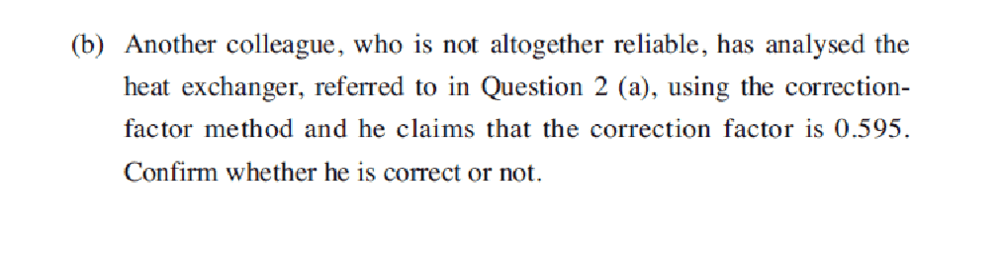Would the equations I posted above prove this wrong please? I worked out that the correction factor=1.68 but have no idea if this is correct.

Mentor
Sorry, the next part of the question asks...
View attachment 241477
Would the equations I posted above prove this wrong please? I worked out that the correction factor=1.68 but have no idea if this is correct.
What is the reciprocal of 1.68?

PCal
0.59! Why have I had to take the reciprocal though?

PCal
Got it! I was doing F=∆Tc x LMTD as opposed to F=∆Tc/LMTD
Thank you for all your help!

•BvU
JohnnyS
Hi, I am working this same question at the moment and I have got the same answers you did for the outlet temperatures and the heat transfer rate of TC2 = 66.25°C and TH2 = 37.5°C, and the transfer rate of 129.15kW.
I am now struggling with the correction factor part of the question. Following the equations given in the lessons which are as you mention above I am not getting to 0.59.

I get :
∆Tc = Φ / UA
129.15 / (0.36 x 14)
∆Tc = 25.625°C
Then

F=∆Tc/LMTD

LMTD = (TC2 - TC1) / In [(TH - TC1) / (TH - TC2)]
= (66.25 - 15) / In [(140 - 15) / (140 - 66.25)]
LMTD = 97.13°C

F=∆Tc/LMTD
25.625 / 97.13
F = 0.264
Please can someone point me in the right direction please as I am going round in circles.